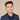# How To Get Absolute Value In Swift

To get the absolute value in Swift, you can use the `abs()` function. Here is a code example:

``````let negativeNumber = -30
let absoluteValue = abs(negativeNumber)
print(absoluteValue) // 30``````

Absolute value is useful when you only care about the magnitude of a number, not whether if it is positive or negative.

If you call `abs()` on a positive number, it will return the same value:

``````print(abs(10))
// 10``````

The `abs()` function also works on `Double` values:

``````let negative = -30.2
let absoluteValue = abs(negative)
print(absoluteValue) // 30.2``````

## Using Magnitude Property

You can use the magnitude property to get the absolute value as well. Here is a code example:

``````let negative = -5
print(negative.magnitude) // 5``````

This also works on `Double` values:

``````let negative2 = -5.5
print(negative2.magnitude) // 5.5``````
If you liked this post and want to learn more, check out The Complete iOS Developer Bootcamp. Speed up your learning curve - hundreds of students have already joined. Thanks for reading!## Eddy Chung

I teach iOS development on ZeroToAppStore.com.